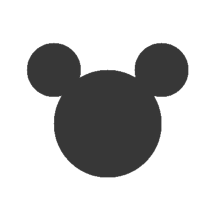# 7.3: Example Drawing

$$\newcommand{\vecs}{\overset { \rightharpoonup} {\mathbf{#1}} }$$ $$\newcommand{\vecd}{\overset{-\!-\!\rightharpoonup}{\vphantom{a}\smash {#1}}}$$$$\newcommand{\id}{\mathrm{id}}$$ $$\newcommand{\Span}{\mathrm{span}}$$ $$\newcommand{\kernel}{\mathrm{null}\,}$$ $$\newcommand{\range}{\mathrm{range}\,}$$ $$\newcommand{\RealPart}{\mathrm{Re}}$$ $$\newcommand{\ImaginaryPart}{\mathrm{Im}}$$ $$\newcommand{\Argument}{\mathrm{Arg}}$$ $$\newcommand{\norm}{\| #1 \|}$$ $$\newcommand{\inner}{\langle #1, #2 \rangle}$$ $$\newcommand{\Span}{\mathrm{span}}$$ $$\newcommand{\id}{\mathrm{id}}$$ $$\newcommand{\Span}{\mathrm{span}}$$ $$\newcommand{\kernel}{\mathrm{null}\,}$$ $$\newcommand{\range}{\mathrm{range}\,}$$ $$\newcommand{\RealPart}{\mathrm{Re}}$$ $$\newcommand{\ImaginaryPart}{\mathrm{Im}}$$ $$\newcommand{\Argument}{\mathrm{Arg}}$$ $$\newcommand{\norm}{\| #1 \|}$$ $$\newcommand{\inner}{\langle #1, #2 \rangle}$$ $$\newcommand{\Span}{\mathrm{span}}$$$$\newcommand{\AA}{\unicode[.8,0]{x212B}}$$

Suppose we want to draw a “Hidden Mickey”, which is an icon that represents Mickey Mouse (see https://en.Wikipedia.org/wiki/Hidden_Mickey). We can use the oval we just drew as the face, and then add two ears. To make the code more readable, let’s use Rectangle objects to represent bounding boxes.

Here’s a method that takes a Rectangle and invokes fillOval:

public void boxOval(Graphics g, Rectangle bb) {
g.fillOval(bb.x, bb.y, bb.width, bb.height);
}


And here’s a method that draws Mickey Mouse:

public void mickey(Graphics g, Rectangle bb) {
boxOval(g, bb);

int dx = bb.width / 2;
int dy = bb.height / 2;
Rectangle half = new Rectangle(bb.x, bb.y, dx, dy);

half.translate(-dx / 2, -dy / 2);
boxOval(g, half);

half.translate(dx * 2, 0);
boxOval(g, half);
}


The first line draws the face. The next three lines create a smaller rectangle for the ears. We translate the rectangle up and left for the first ear, then to the right for the second ear. The result is shown in Figure 16.3.1.Figure $$\PageIndex{1}$$: A “Hidden Mickey” drawn using Java graphics.

You can read more about Rectangle and translate in Chapter 10. See the exercises at the end of this appendix for more example drawings.

This page titled 7.3: Example Drawing is shared under a CC BY-NC-SA 3.0 license and was authored, remixed, and/or curated by Allen B. Downey (Green Tea Press) .